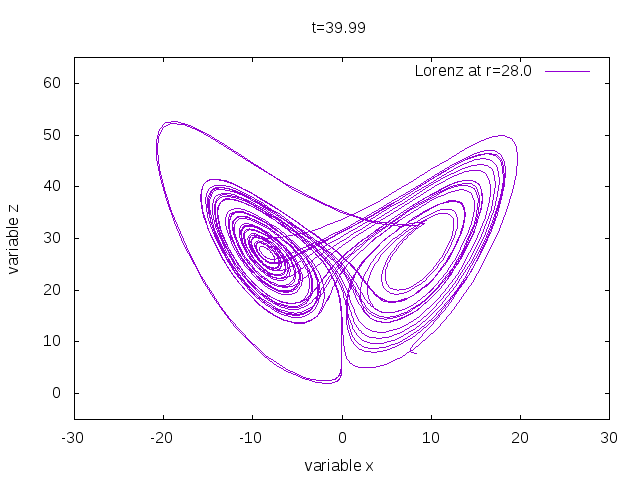# Lorenz system

## An interactive simulation of a chaotic attractor

created by Hendrik Wernecke

— summer term 2018 —
The Lorenz system was defined by Lorenz  and is very important.
Contents

### Simulation

time $t =$0.00
$\rho=$

### Usage

This simulation is damn hard to use. Press the START button and it runs. Press STOP and it stops.

### Theory

This is the Lorenz system: \begin{align} \dot{x} &= \sigma(y-x)\\ \dot{y} &= x(\rho-z)-y\\ \dot{z} &= xy-\beta z\,. \end{align} We use $\beta=8/3$ and $\sigma=10$ and keep $\rho$ as a parameter The syste has the following fixed points \begin{align} \mathbf{v}_\text{o} &= (0,0,0)\\ \mathbf{v}_{1,2} &= \left(\pm\sqrt{\beta(\rho-1)},\pm\sqrt{\beta(\rho-1)},\rho-1\right) \end{align} which are also indicated on the canvas.

For $r=28$ the Lorenz system is chaotic and then it looks likeFigure: The Lorenz butterfly.

### Code & documentation

Here I want to show some parts of the JavaScript code that I am fond of:
• solving the Lorenz ODE by Euler integration
• the core drawing function looks like this

### Acknowledgements, authors & contact

Acknowledgements The author would like to thank everyone.

About the authors Hendrik Wernecke is a PhD student in the group of Prof. C. Gros at Goethe University Frankfurt (Main).

Contact For questions, suggestions or comments on this simulation, please contact click to show email

### References & further reading


: Deterministic nonperiodic flow., J. Atmos. Sci. 20(2) 1963.

Legal note: We do not take any responsibility for
the content of the webPages linked here-in.